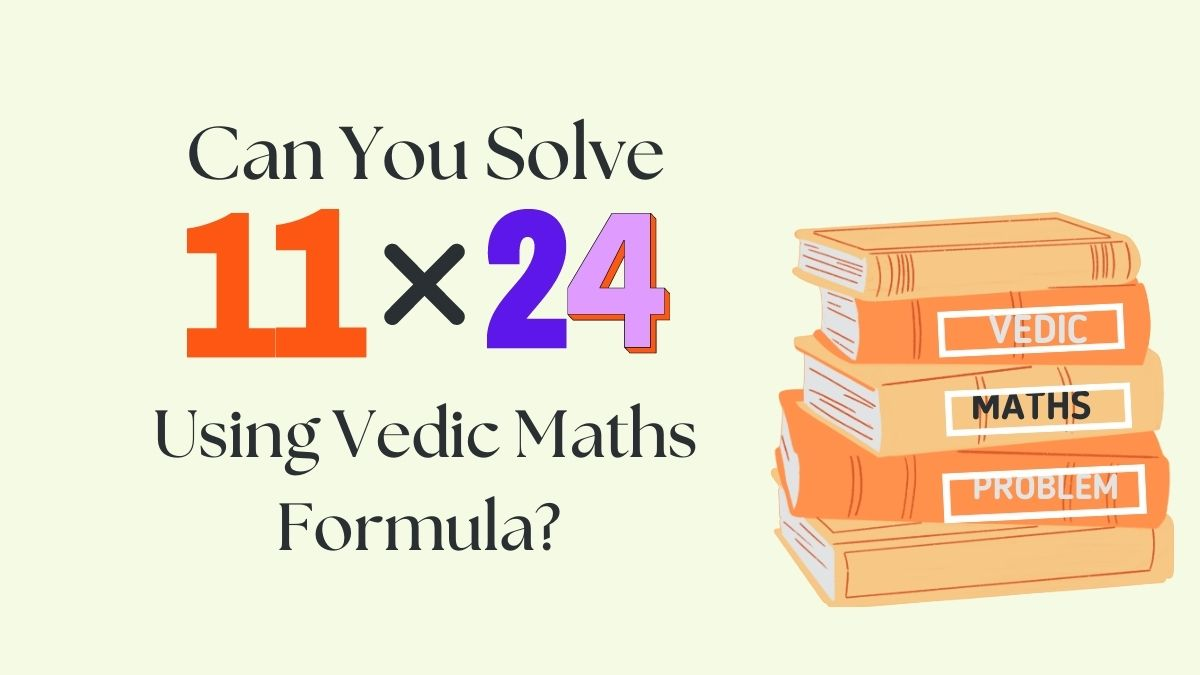# Vedic Math Puzzle: Are You Skilled Enough To Solve Basic Multiplication Using Vedic Formula in 27 Seconds?

Vedic Math Puzzle: This Vedic math puzzle demands your quantitative and cognitive skills. Do you think you will be able to solve this one?Try this Vedic Math Puzzle

Vedic Math Puzzle: A Vedic math puzzle is a mathematical problem or equation that utilizes techniques from Vedic mathematics, an ancient Indian system, to find solutions in a quicker and more efficient manner.

Vedic math puzzles are important as they promote critical thinking, problem-solving skills, and mental agility while utilizing ancient mathematical techniques, enhancing overall mathematical aptitude and fostering an appreciation for Vedic mathematics. Here in this equation, your task is to find the product of two units using one of the formulae of Vedic Maths.

Look for the equation below:

Do You Think You Will Be Able To Solve?

Don’t Say It’s Not Your Cup Of Tea!

## What is Vedic Math?

Vedic math multiplication is a set of techniques derived from ancient Indian mathematics that offer efficient and alternative methods for multiplying numbers. These methods can simplify complex multiplication problems and make calculations faster and easier.

History and Sutras of Vedic Math

Hey Genius…

You are Losing…

Solving Vedic math equations offers several benefits. It enhances mental calculation skills, improves speed and accuracy in mathematical operations, promotes logical thinking and problem-solving abilities, and provides alternative methods for approaching complex calculations, making mathematics more enjoyable and accessible.

Tick…

Tock…

Tick…

I am sure you have a digit. Just tally your steps and answers with the below picture.

Understand the steps here:

Step 1: 1 * 4 = 4 (last digit)

Step 2: 4 * 1 + 1 * 2 = 6

Step 3: 1 * 2 = 2 (first digit)

Therefore, the correct answer is 264.

This two-digit multiplication equation is solved using the Sutra (formula) of Urdhva-Tiryagbyham. It is a Vedic math technique that involves vertical and crosswise multiplication, enabling efficient multiplication of multi-digit numbers by breaking them down into simpler computations.

You did Well. To learn more about Vedic Maths and its different Sutras, keep scrolling back to Jagran Josh.

Also Try:

Only 1% Of Genius Can Find The Product of The Two Digits Below With Vedic Sutra in 19 Seconds. Hurry Up!

Check Your IQ Level By Finding The Odd Pair Of Red Heels In 7 Seconds. Good Luck!

Help Rebecca to find the odd pair of earrings hidden in the picture within 7 seconds. Hurry Up!

Get the latest General Knowledge and Current Affairs from all over India and world for all competitive exams.
खेलें हर किस्म के रोमांच से भरपूर गेम्स सिर्फ़ जागरण प्ले पर